INDIRECT函数的工作方式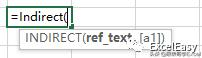INDIRECT(ref_text,[a1])

=INDIRECT("A1")

=INDIRECT(A2)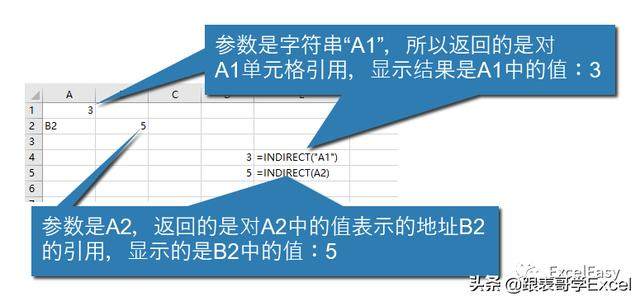=SUM(INDIRECT("A1:A20"))

=SUM(A1:A20)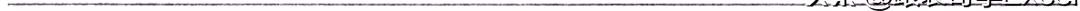INDIRECT函数的用法举例

=SUM(INDIRECT("A1:A"&ROW()))

=INDIRECT("'[你的Excel文件.xlsx]你的工作表'!你的地址")

=INDIRECT("'[" & C1 &"]Sheet1'!A1:A100")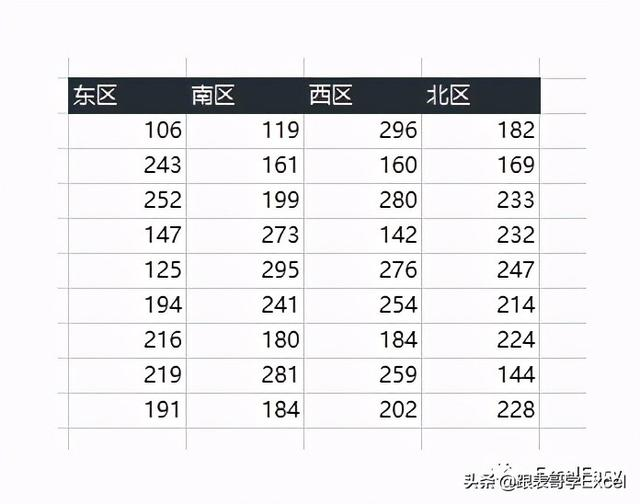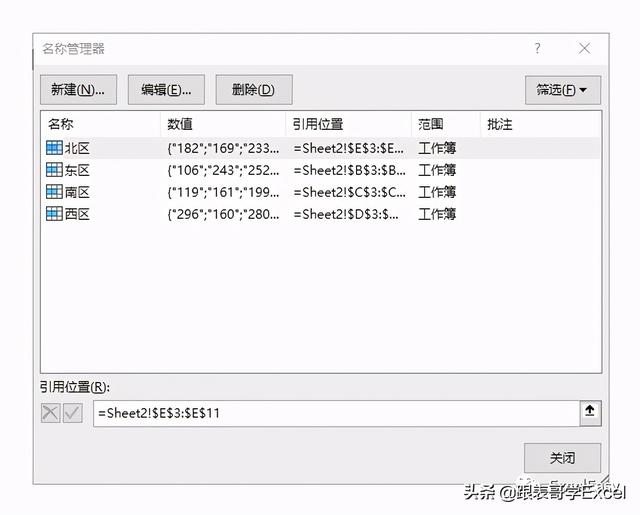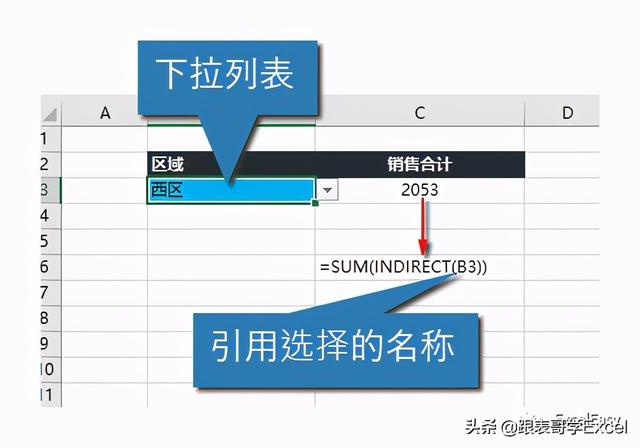=INDIRECT(B2)